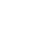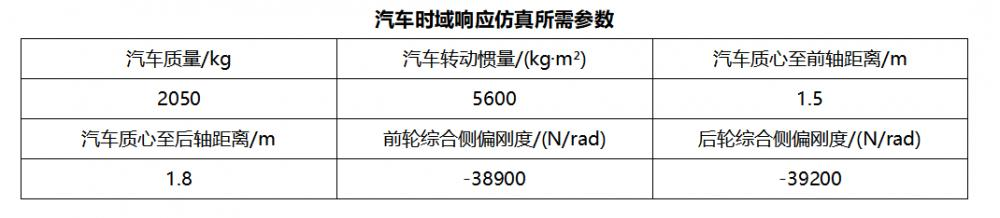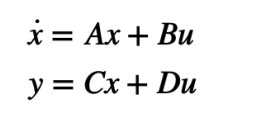>> m=2050;Iz=5600;a=1.5;b=1.8;L=3.3;

>> k1=-38900;k2=-39200;

>> u= [20 30 40];

>> a11 = (k1 + k2)/m./u;

>> a12 = (a*k1 - b*k2 -m.*u.^2)/m./u.^2;

>> a21 = (a*k1 - b*k2)/Iz;

>> a22=(a^2*k1 + b^2*k2)/Iz./u;

>> b11 = -k1/m./u;

>> b21 = -a*k1/Iz;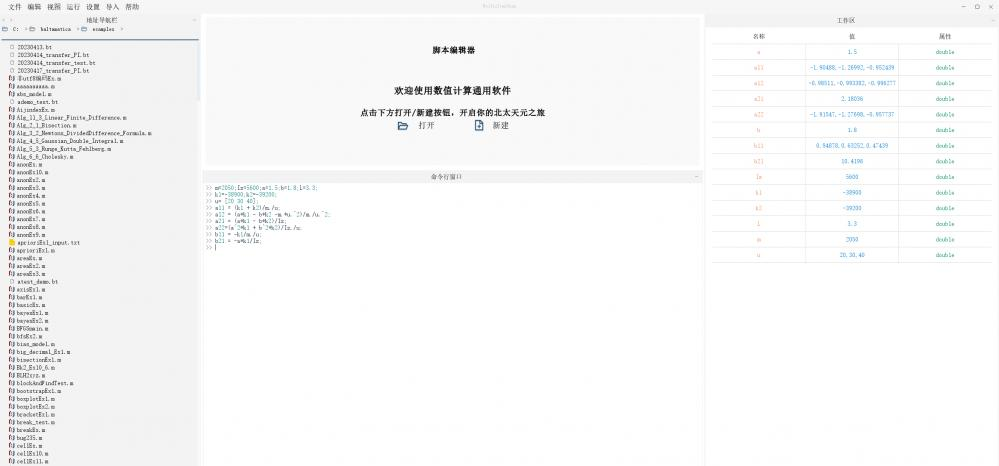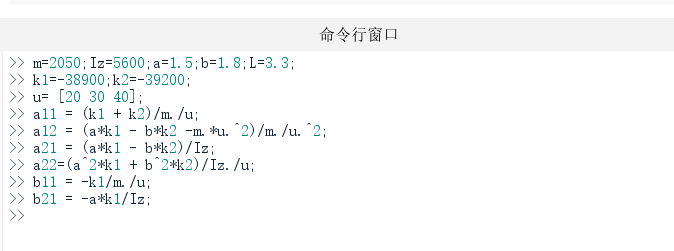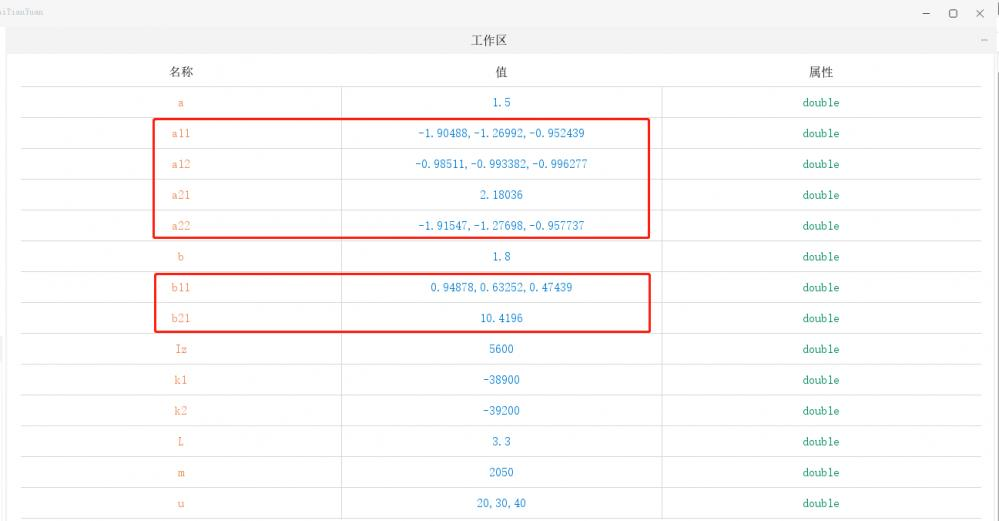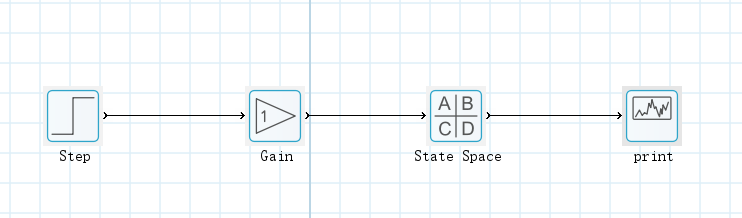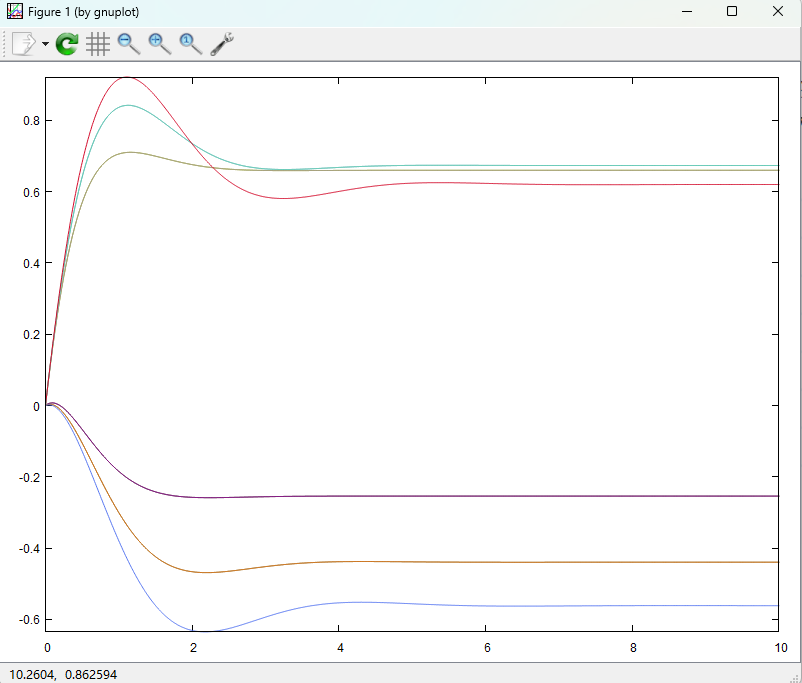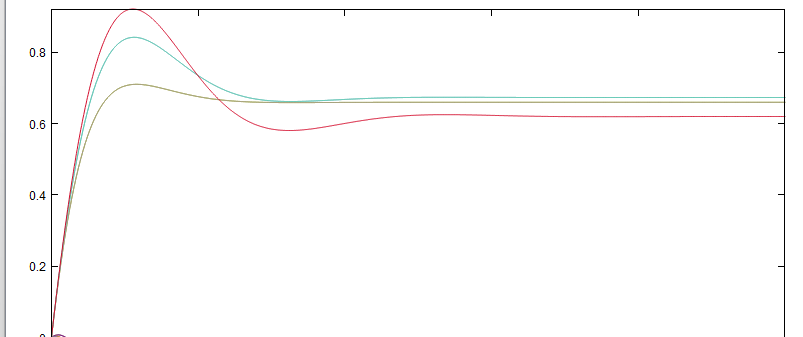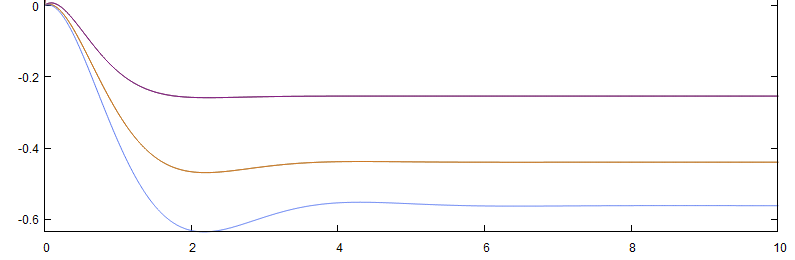255 0 0 收藏 回复

## 回复

### 社区牛人